Photo of the month – May 2006 [German version]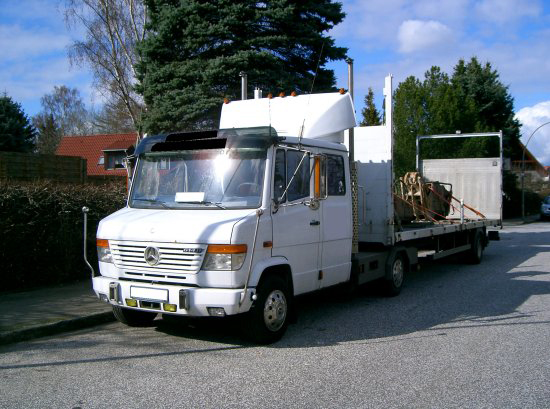Figure 1 [Achim Kock]

On its trailer, the truck is carrying a mobile container for flammable liquids, a hydraulic breaker and a pulverizer.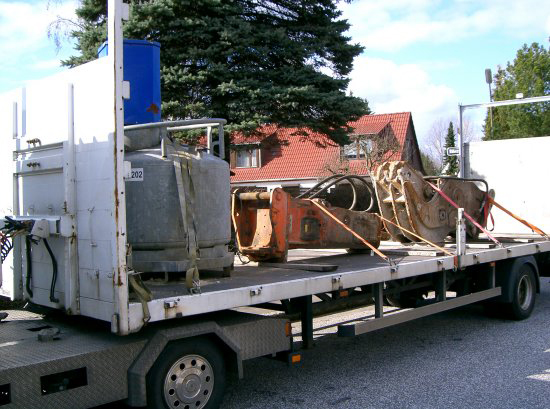Figure 2 [Achim Kock]

The load securing measures taken (friction securing and tie-downs) are virtually without effect either horizontally or laterally. A close look at Figures 3 through 5 below gives rise to the following list of deficiencies: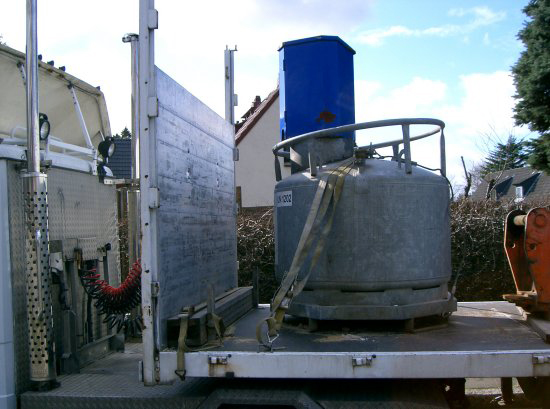Figure 3 [Achim Kock]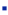Figure 3 clearly shows that the loading area is heavily contaminated with sand. For the combination of wood on loading area, a coefficient of friction of µ = 0.3 can normally be assumed. The sand on the surface would probably reduce this to µ = 0.15. This is compounded by the fact that the hazardous goods container is secured by just one tie-down; at least two should be used. The securing effect of the belt will not be more than 75 daN. It is only to be hoped that the container does not weigh more than 115 kg with or without contents.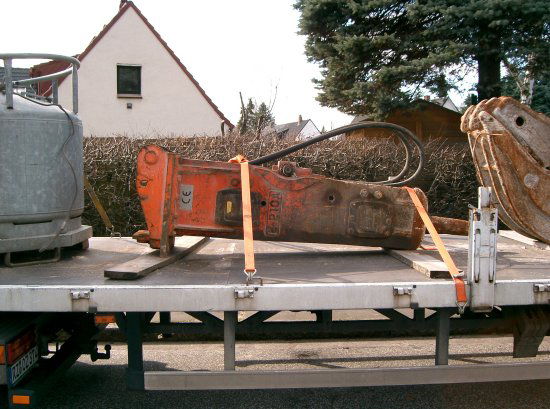Figure 4 [Achim Kock]Figure 4 shows the hydraulic breaker resting on wooden boards and tied down with two belts. One of the hooks of one of the tie-downs is attached to the chassis of the trailer, and only the tip of the hook engages the chassis. This means that the hook is no longer subjected to a tensile load, but to a bending load. The following calculation shows that the securing force of the tie-downs is not sufficient to secure the load.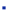The weight of the load is approx. 1 metric ton (quite possibly considerably more); this results in the following load weight force:
1,000 kg x 1 g [1 g = 9.81 m/s² or rounded to 10 m/s²] = 10,000 N = 1,000 daNThe tie-down belts have a lashing capacity (LC) of 2,500 daN, which means that prestressing in a straight line (normal ratchet) is 250 daN (0.1 x LC) for each end of the belt, i.e. prestressing of 500 daN per belt under ideal conditions.
[Simply doubling the prestressing value is an ideal value for the purposes of calculation. In reality, due to the friction between the belt and the load, the prestressing is only partially transferred from the side on which the tightener acts to the other side. Edge protector corners can reduce this friction.]The coefficient of friction for the combination of wood on a sand-contaminated loading area is generously assumed to be µ = 0.2.The following longitudinal securing force is required:
80% of the load weight force –> 1,000 daN x 0.8 g = 800 daNThe following lateral securing force is required:
70% of the load weight force as the load is liable to tip (otherwise, this would be 50%) –> 1,000 daN x 0.7 g = 700 daNSecuring force as a result of friction (µ = 0.2): 1,000 daN x 0.2 = 200 daNSecuring force/effect of the tie-down lashings:
 – 500 daN prestressing per belt (see above) – If a tie-down is attached at an angle, the securing force of the belt is reduced. In this case, the lashing angle is assumed to be 45°, i.e. the longitudinal efficiency of the belt is 71% (sin45°=0.71). The lateral efficiency can still be assumed to be 100%. – Longitudinal: 2 belts x securing force (see above) x friction = 2 x (500 daN x 0.71) x 0.2 = 142 daN – Lateral: 2 belts x securing force (see above) x friction = 2 x 500 daN x 0.2 = 200 daNShortfall in longitudinal securing force:
800 daN – 200 daN – 142 daN = 458 daNShortfall in lateral securing force:
700 daN – 200 daN -200 daN = 300 daNThe calculation above was extremely generous. It can be assumed that the weight of the load is higher and the coefficient of friction lower.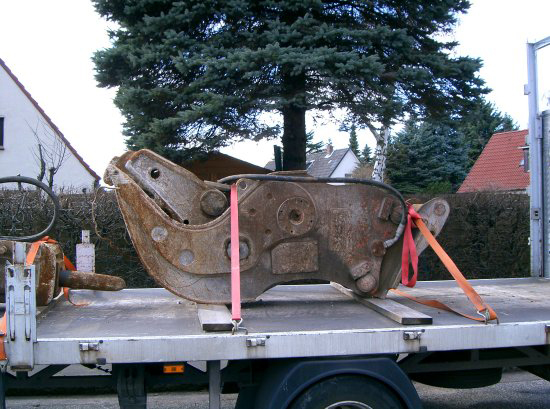Figure 5 [Achim Kock]The load weight of the pulverizer (Figure 5) was in all probability more than 2 metric tons and was secured in the same way as the hydraulic breaker. The load does not appear to be liable to tip, but calculations carried out in the same way as above quickly show that the load securing measures were wholly inadequate.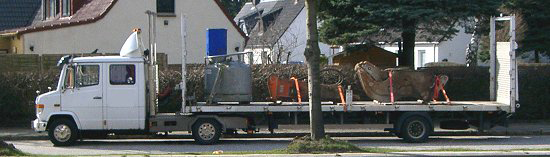Figure 6 [Achim Kock]

Possible improvements:Increase the friction by cleaning the loading area and using friction-enhancing materials (nonslip mats).Securing of the load by means of a tight fit (direct securing), e.g. lashings to the sides, lashings or head loops to the front and back or direct securing using wooden bracing against the borders of the loading area or against stanchions. To achieve this, however, a suitable vehicle would have to be used.

Deficient load securing can be a potentially fatal hazard, as the articles for the months November 2002, November 2003 and November 2005 strikingly illustrate.

Back to beginning